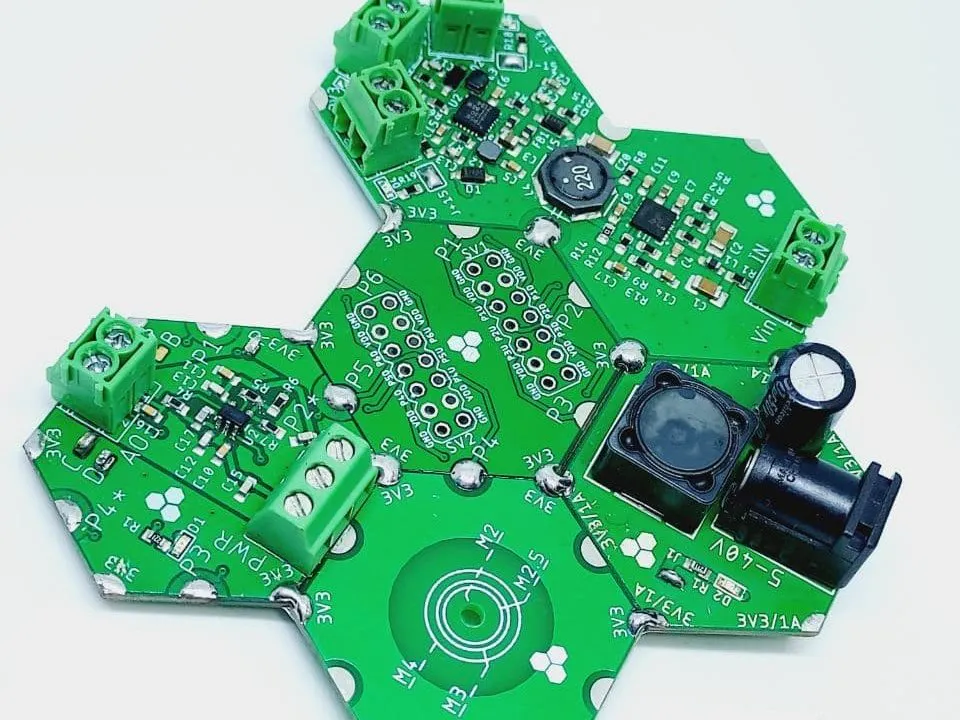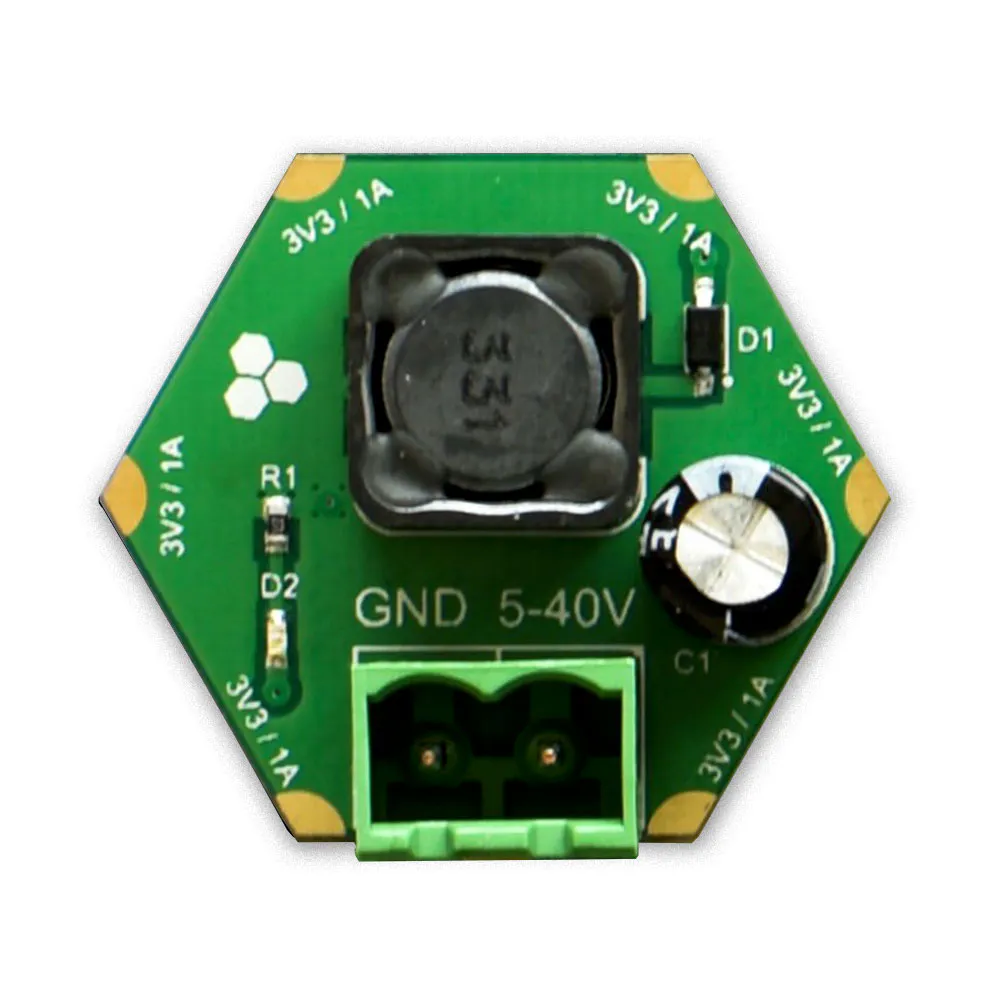Published © MIT

# Hexabitz Function Generator

Function Generator Using Hexabitz Modules

IntermediateProtip5 hours64## Things used in this project

### Hardware componentsHexabitz 3.3V/1A DC-DC Power Supply Module (H03R00)
×1
 Hexabitz Isolated Bipolar +/-15V DC Power Supply
×1
 Hexabitz Bipolar 10V, 12-bit Analog Output
×1
 Hexabitz H00R2 Vertical Extension
×1

### Hand tools and fabrication machines10 Pc. Jumper Wire Kit, 5 cm Long

Read more

## Code

### H15R0x.uvprojx

C Header File
```/**
******************************************************************************
* File Name          : main.c
* Description        : Main program body
******************************************************************************
MODIFIED by Hexabitz for BitzOS (BOS) V0.2.3 - Copyright (C) 2017-2020 Hexabitz
All rights reserved
*/

/* Includes ------------------------------------------------------------------*/
#include "BOS.h"
#include "BOS_adc.h"
#include "math.h"
/* Private variables ---------------------------------------------------------*/
uint32_t var_0 = 0 ,var_1=0 ,var=0 , wave_absolute = 0 ,wave_freq = 0 ,wave_form=0  ;
uint32_t wave_mode = 0 ; // mode = 0 => Off , mode = 1 => sine wave , mode = 2 =>  wave,mode = 3 => sawtooth wave
uint32_t wave_mode_2 = 0 , i=0 , volt_shift=500;
uint32_t max_val = 1500 , value_before_out = 0 ;
uint32_t last_mode, freq_before_out = 100;
uint32_t compare_value=0;
float wave;
float wave2;
float wave_absolute_100 ;
float wave_freq_100 ;

TIM_HandleTypeDef htim2;
/* Private function prototypes -----------------------------------------------*/
void SystemClock_Config(void);
static void MX_TIM2_Init(void);
void sine_wave(void);
void Traingle_wave(void);
void sawtooth_wave(void);

/* Main functions ------------------------------------------------------------*/

int main(void)
{
/* MCU Configuration----------------------------------------------------------   */
/* Reset all peripherals, Initializes the Flash interface and the Systick. */
HAL_Init();

/* Configure the system clock */
SystemClock_Config();

/* Initialize all user peripherals */

/* Initialize BitzOS */
BOS_Init();
ADC_Init();

MX_TIM2_Init();
HAL_TIM_Base_Start_IT(&htim2);

/* Call init function for freertos objects (in freertos.c) */
MX_FREERTOS_Init();
/* Start scheduler */
osKernelStart();

/* We should never get here as control is now taken by the scheduler */
/* Infinite loop */
while (1)
{

}
}
/*-----------------------------------------------------------*/

/* User Task */
void UserTask(void * argument)
{
AddPortButton(MOMENTARY_NO,P1);
AddPortButton(MOMENTARY_NO,P3);
AddPortButton(MOMENTARY_NO,P4);
SetButtonEvents( P1, 1,0, 0,0 , 0,  0, 0, 0,0);
SetButtonEvents( P4, 1,0, 0,0, 0,  0, 0, 0,0);
SetButtonEvents( P3, 1,0, 0, 0, 0,  0, 0, 0,0);
IND_ON();
HAL_Delay(1000);
AnalogPercentage (75);
/* Infinite loop */
for(;;)
{
HAL_ADC_Start(&hadc);
if(HAL_ADC_PollForConversion(&hadc,5)==HAL_OK)
{
var_0 = HAL_ADC_GetValue(&hadc);
}

if(HAL_ADC_PollForConversion(&hadc,5)==HAL_OK)
{
var_1 = HAL_ADC_GetValue(&hadc);

}
HAL_ADC_Stop(&hadc);

wave_absolute_100 = var_0 *100 / 255 ;
wave_absolute_100 =wave_absolute_100 / 100 ;
wave_freq_100 = var_1 *100 / 255 ;
wave_freq_100 =wave_freq_100 / 100 ;
wave_freq_100 = wave_freq_100 *500	;
freq_before_out  = (uint32_t) wave_freq_100;
//	htim2.Init.Period =freq_before_out;

Delay_ms(100);
}
}
//------------------------------------//
void HAL_TIM_PeriodElapsedCallback(TIM_HandleTypeDef *htim)
{
/* Prevent unused argument(s) compilation warning */
UNUSED(htim);
if(freq_before_out<10)freq_before_out=10;
__HAL_TIM_SET_AUTORELOAD(&htim2,freq_before_out);
wave_mode_2 = wave_mode ;
if(wave_mode==last_mode && wave_mode!=0)
{
var=var+1;
//-------------------------------
value_before_out= (uint32_t) ( ((wave[var] * wave_absolute_100)+volt_shift) / 10) ;
if (value_before_out>100)value_before_out=100;
AnalogPercentage (value_before_out);
//-------------------------------
}
else if (wave_mode!=last_mode)
{
last_mode	=wave_mode;
volt_shift=500;
var=0;
//AnalogPercentage (50);
}

if(var>=99)
{
var=0;
}

//HAL_TIM_Base_Start_IT(&htim2);
/* NOTE : This function should not be modified, when the callback is needed,
the HAL_TIM_PeriodElapsedCallback could be implemented in the user file
*/
}
//=======================================
void buttonClickedCallback(uint8_t port)
{
if(port==P3)
{
if(volt_shift<980)volt_shift= volt_shift+ 20;
}
else if(port==P4)
{
if(volt_shift>=20)volt_shift= volt_shift- 20;
}
else if(port==P1)
{
wave_mode=wave_mode+ 1 ;
if(wave_mode>3)
{
wave_mode =0 ;
}

if(wave_mode==1){
sine_wave();

}
else if(wave_mode==2)
{
Traingle_wave();

}
else if (wave_mode==3)
{
sawtooth_wave();

}

}

}
//--------------------------------------

void sine_wave(void)
{
for(i=0;i<=99;i++)
{
wave[i]=(sin(2*3.14*i/100) * 500)+500;
}
return;
}
//--------------------------------------
void Traingle_wave()
{
for(i=0;i<=49;i++)
{
wave[i]=(i * 1000 /100)+250;
}
//--------------------------------------
for(i=50;i<=99;i++)
{
wave[i]=((100-i) * 1000 /100)+250;
}

return;
}
//--------------------------------------
void sawtooth_wave()
{
for(i=0;i<=99;i++)
{
wave[i]=(i * 500 /100)+250;
}
}
//================================
static void MX_TIM2_Init(void)
{
/* USER CODE BEGIN TIM2_Init 0 */

/* USER CODE END TIM2_Init 0 */

TIM_ClockConfigTypeDef sClockSourceConfig = {0};
TIM_MasterConfigTypeDef sMasterConfig = {0};

/* USER CODE BEGIN TIM2_Init 1 */

/* USER CODE END TIM2_Init 1 */
htim2.Instance = TIM2;
htim2.Init.Prescaler = 480;
htim2.Init.CounterMode = TIM_COUNTERMODE_UP;
htim2.Init.Period = 100;
htim2.Init.ClockDivision = TIM_CLOCKDIVISION_DIV1;
htim2.Init.AutoReloadPreload =  0x00000000U;
if (HAL_TIM_Base_Init(&htim2) != HAL_OK)
{

}
sClockSourceConfig.ClockSource = TIM_CLOCKSOURCE_INTERNAL;
if (HAL_TIM_ConfigClockSource(&htim2, &sClockSourceConfig) != HAL_OK)
{

}
sMasterConfig.MasterOutputTrigger = TIM_TRGO_RESET;
sMasterConfig.MasterSlaveMode = TIM_MASTERSLAVEMODE_DISABLE;
if (HAL_TIMEx_MasterConfigSynchronization(&htim2, &sMasterConfig) != HAL_OK)
{

}
/* USER CODE BEGIN TIM2_Init 2 */
/* USER CODE END TIM2_Init 2 */
}
/*-----------------------------------------------------------*/
/************************ (C) COPYRIGHT HEXABITZ *****END OF FILE****/
```

## Credits

### Hussein Jawish

1 project • 1 follower

### Mahmoud Mardnly

8 projects • 4 followers